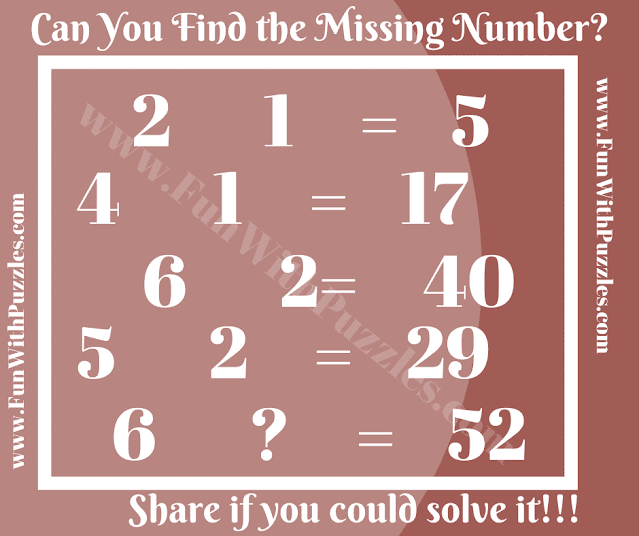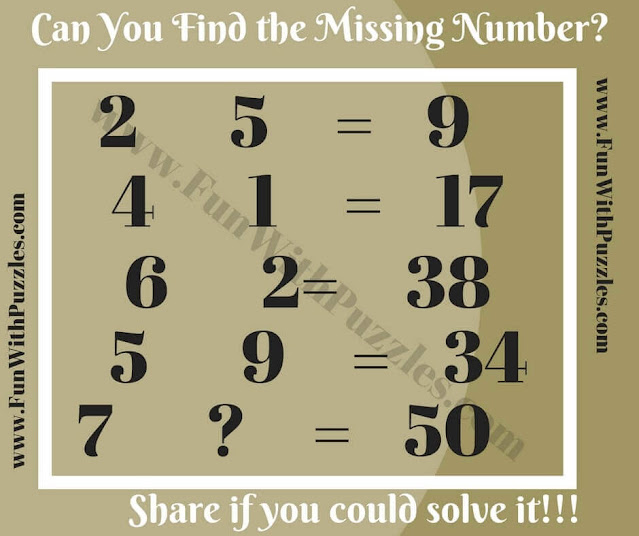Get ready to give your intellect a workout with our captivating collection of Maths Logic Puzzles. These brain teasers bring together the fascinating worlds of Mathematics and Logical Reasoning, creating an engaging challenge that will keep you hooked. In each puzzle, you'll encounter a series of equations that might initially seem mathematically unconventional. However, there's a twist – these equations follow a hidden logical pattern. Your task is to unearth this underlying connection, ultimately leading you to crack the code and uncover the missing number in the final equation.1. Crack the Code Challenges: Maths Logic Puzzle

Embrace the joy of deciphering intricate relationships and showcasing your mathematical prowess. These brain teasers not only require a keen eye for detail but also the ability to apply logical deduction. As you navigate through the challenges, you'll develop a deeper understanding of how logic and mathematics can intersect in unexpected ways.

Unlock your analytical potential by exploring the realm of Maths Logic Puzzles. Delve into each puzzle, unravel the coded patterns, and triumphantly solve the riddles. Whether you're a puzzle enthusiast, a budding logician, or someone looking to exercise their cognitive faculties, these puzzles offer an enjoyable and enriching experience. Get ready to embark on a journey of exploration, challenge, and growth as you dive into the captivating world of Maths Logic Puzzles.2. Crack the Code Challenges: Maths Logic Puzzle3. Crack the Code Challenges: Maths Logic Puzzle4. Crack the Code Challenges: Maths Logic Puzzle5. Crack the Code Challenges: Maths Logic Puzzle

You must try the following Maths and Logic Puzzles and Brain Teasers to crack your brain

## List of Logical and Mathematical Brain Teasers

1. Mathematical Logic Brain Cracking Problems: These contain Mathematical and Logical Brain Teasers to challenge your brain.

2. Brain Wiring Test: It contains a mix of Mathematical Equation Puzzles, Mathematical Sequence Puzzles, and Logical Brain Teasers.

3. Interesting Brain Teasers to Challenge your Mind: It contains a mix of Logical Brain Teasers and Visual Picture Puzzles to challenge your mind.

4. Easy but Brain-teasing Math Problems for Kids: These are very interesting easy but challenging Maths problems in which your challenge is to add Mathematical operators in Math Equations to correct these equations.

Answers to these Maths Logic Brain Teasers are given below. Use these answers to check your solution. In case you have a different logic to solve these puzzles, do post your explanation and answer in the comments.
1) 14

2) 4

3) 3

4) 20

5) 1